Construction of a circle when its radius is known

Chapter 14 Class 6 Practical Geometry
Concept wise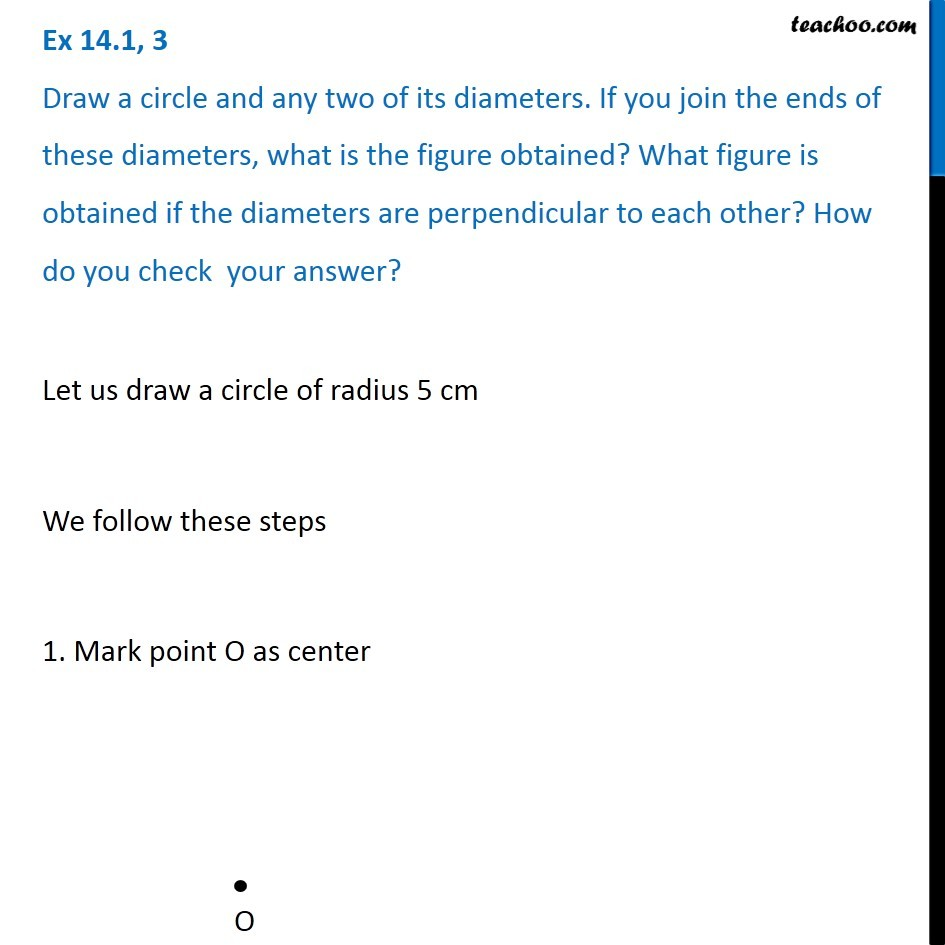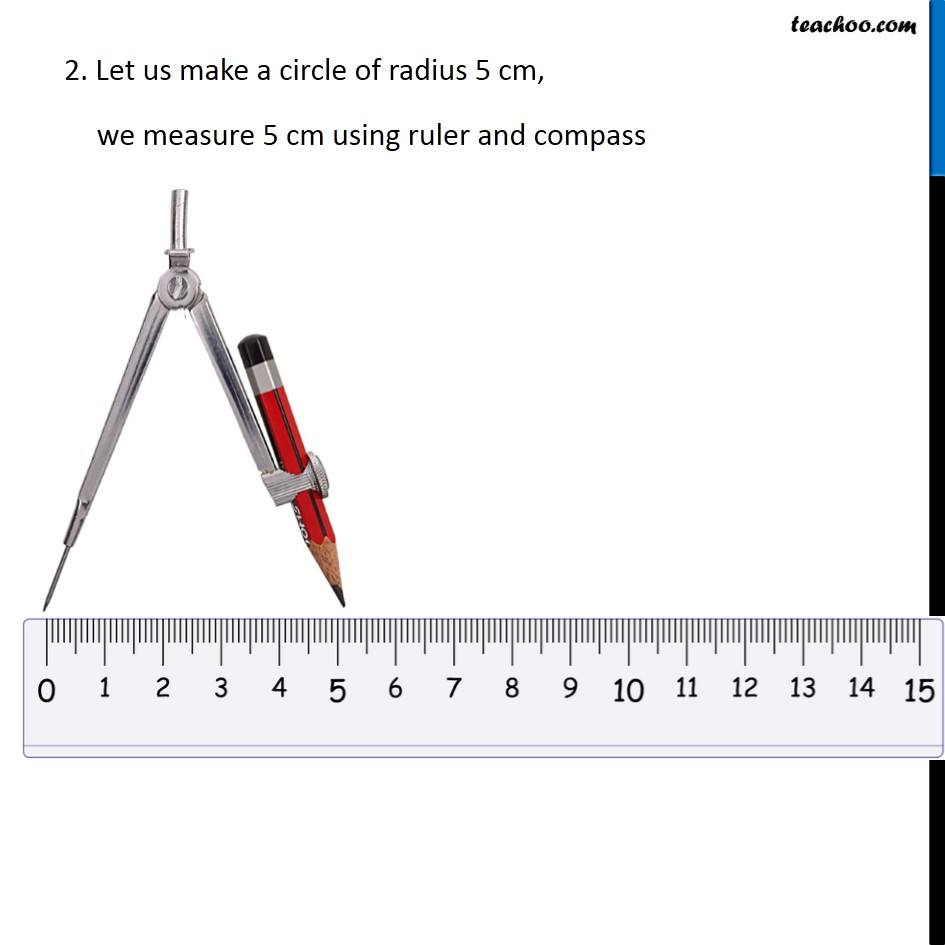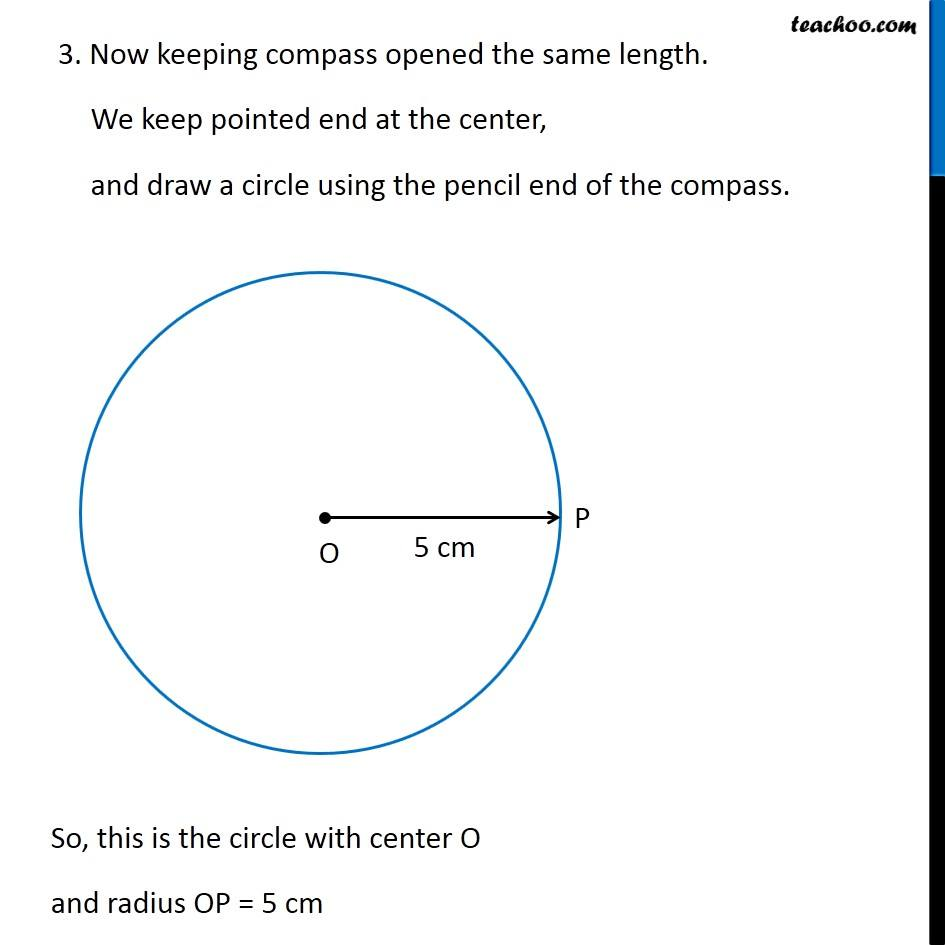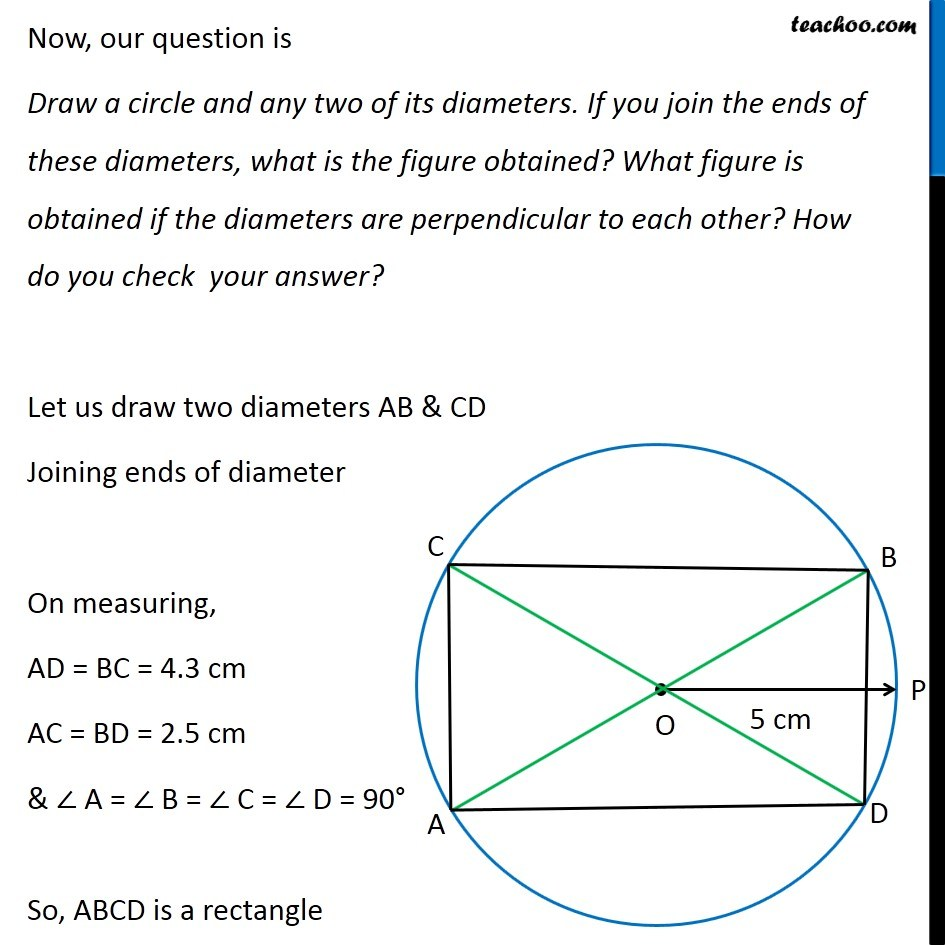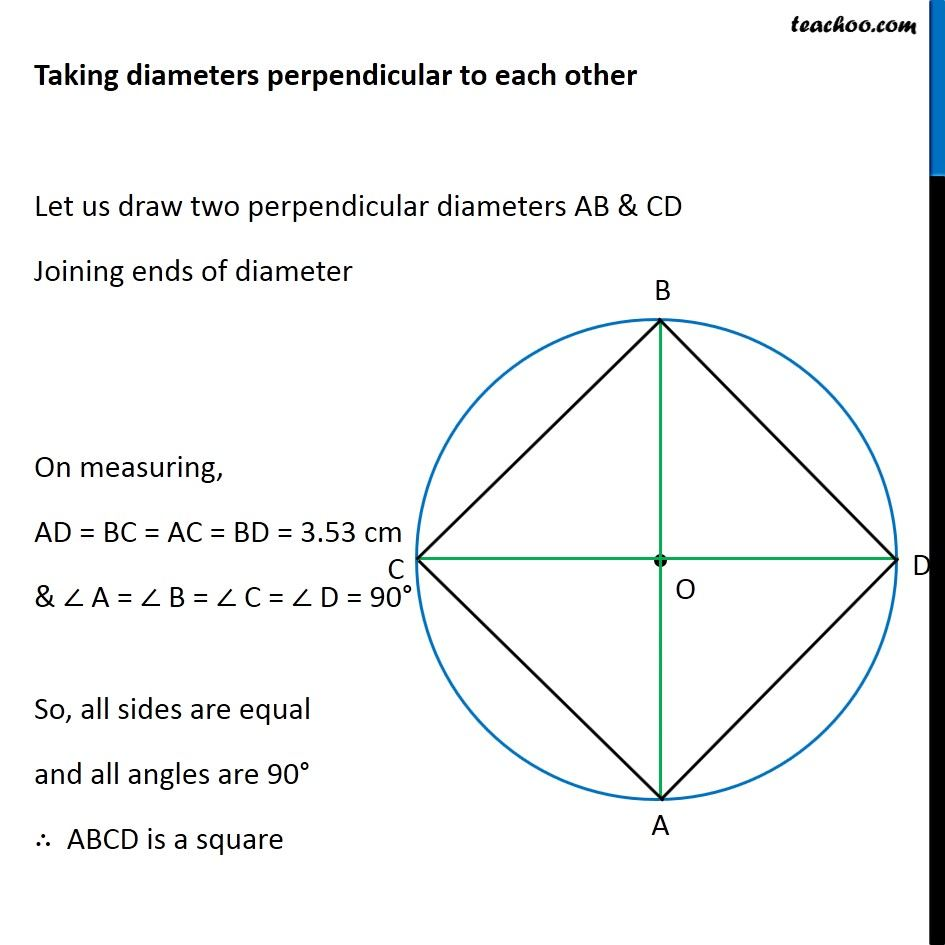### Transcript

Ex 14.1, 3 Draw a circle and any two of its diameters. If you join the ends of these diameters, what is the figure obtained? What figure is obtained if the diameters are perpendicular to each other? How do you check your answer? Let us draw a circle of radius 5 cm We follow these steps 1. Mark point O as center 2. Let us make a circle of radius 5 cm, we measure 5 cm using ruler and compass 3. Now keeping compass opened the same length. We keep pointed end at the center, and draw a circle using the pencil end of the compass. So, this is the circle with center O and radius OP = 5 cm Now, our question is Draw a circle and any two of its diameters. If you join the ends of these diameters, what is the figure obtained? What figure is obtained if the diameters are perpendicular to each other? How do you check your answer? Let us draw two diameters AB & CD Joining ends of diameter On measuring, AD = BC = 4.3 cm AC = BD = 2.5 cm & ∠ A = ∠ B = ∠ C = ∠ D = 90° So, ABCD is a rectangle Taking diameters perpendicular to each other Let us draw two perpendicular diameters AB & CD Joining ends of diameter On measuring, AD = BC = AC = BD = 3.53 cm & ∠ A = ∠ B = ∠ C = ∠ D = 90° So, all sides are equal and all angles are 90° ∴ ABCD is a square